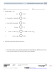Lesson 30: Special Lines in Triangles M1 Lesson 30

Transcription

Lesson 30: Special Lines in Triangles M1 Lesson 30
Lesson 30
NYS COMMON CORE MATHEMATICS CURRICULUM
M1
GEOMETRY
Lesson 30: Special Lines in Triangles
Classwork
Opening Exercise
In
at the right, is the midpoint of ̅̅̅̅
is the midpoint of ̅̅̅̅ , and
̅̅̅̅
is the midpoint of
. Complete each statement below.
̅̅̅̅ is parallel to
and measures
the length of
.
̅̅̅̅ is parallel to
and measures
the length of
.
̅̅̅̅ is parallel to
and measures
the length of
.
Discussion
In the previous two lessons, we proved that (a) the midsegment of a triangle is parallel to the third side and half the
length of the third side and (b) diagonals of a parallelogram bisect each other. We use both of these facts to prove the
following assertion:
All medians of a triangle are
. That is, the three medians of a triangle (the segments connecting
each vertex to the midpoint of the opposite side) meet at a single point. This point of concurrency is called the
, or the center of gravity, of the triangle. The proof will also show a length relationship for
each median: The length from the vertex to the centroid is
the length from the centroid to the
midpoint of the side.
Lesson 30:
Date:
Special Lines in Triangles
10/15/14
© 2014 Common Core, Inc. Some rights reserved. commoncore.org
S.163
Lesson 30
NYS COMMON CORE MATHEMATICS CURRICULUM
M1
GEOMETRY
Example 1
Provide a valid reason for each step in the proof below.
Given:
Prove:
with , , and
the midpoints of sides
The three medians of
(1) Draw
and
,
(5)
,
(7)
; label their intersection as point .
and
as point
and the midpoint of
,
is a parallelogram,
(8)
and
(9)
and
(10)
, respectively.
,
(4)
(6)
, and
meet at a single point.
(2) Construct and label the midpoint of
as point .
(3)
,
,
,
and
Lesson 30:
Date:
,
Special Lines in Triangles
10/15/14
© 2014 Common Core, Inc. Some rights reserved. commoncore.org
S.164
Lesson 30
NYS COMMON CORE MATHEMATICS CURRICULUM
M1
GEOMETRY
(11)
and
,
(12) We can complete steps (1)–(11) to include the median from ; the third median, ̅̅̅̅ , passes through point , which
divides it into two segments such that the longer part is twice the shorter.
(13) The intersection point of the medians divides each median into two parts with lengths in a ratio of 2:1; therefore, all
medians are concurrent at that point.
The three medians of a triangle are concurrent at the
, or the center of gravity. This point of
concurrency divides the length of each median in a ratio of
; the length from the vertex to the
centroid is
the length from the centroid to the midpoint of the side.
Example 2
In the figure to the right,
,
,
. Find each of the following measures.
a.
b.
c.
d.
e.
f.
Lesson 30:
Date:
Special Lines in Triangles
10/15/14
© 2014 Common Core, Inc. Some rights reserved. commoncore.org
S.165
Lesson 30
NYS COMMON CORE MATHEMATICS CURRICULUM
M1
GEOMETRY
Example 3
In the figure to the right,
̅̅̅̅ , respectively. If
is reflected over ̅̅̅̅ to create
, prove that
.
Lesson 30:
Date:
. Points , , and
are midpoints of ̅̅̅̅ , ̅̅̅̅ , and
Special Lines in Triangles
10/15/14
© 2014 Common Core, Inc. Some rights reserved. commoncore.org
S.166
Lesson 30
NYS COMMON CORE MATHEMATICS CURRICULUM
M1
GEOMETRY
Problem Set
Ty is building a model of a hang glider using the template below. To place his supports accurately, Ty needs to locate the
center of gravity on his model.
1.
Use your compass and straightedge to locate the center of gravity on Ty’s model.
2.
Explain what the center of gravity represents on Ty’s model.
3.
Describe the relationship between the longer and shorter sections of the line segments you drew as you located the
center of gravity.
Lesson 30:
Date:
Special Lines in Triangles
10/15/14
© 2014 Common Core, Inc. Some rights reserved. commoncore.org
S.167

Lesson 2: The Area of Right Triangles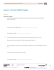Lesson 3: The Area of Acute Triangles Using Height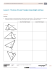Algebra with shapes How to . . .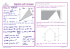Solve the problems below to answer the riddle: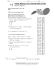The Midpoint Formula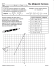Lesson 18: Determining Surface Area of Three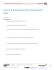Lesson 5: The Area of Polygons Through Composition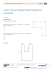Lesson 26: Percent of a Quantity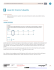5.1 Midsegment Theorem and Coordinate Proof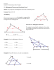Lesson 24: Applications of Systems of Equations and Inequalities M1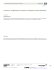Lesson 17: Equations Involving Factored Expressions M1 Lesson 17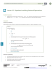1-2 Practice A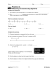Time: 3 to 3 ½ hours M.M.:90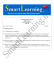Lesson 14 - EngageNY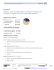Lesson 19: Surface Area and Volume in the Real World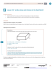4/21/15 11:17 AM Cool Math 4 Kids - Coloring Book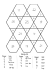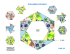Lesson 21: Writing and Evaluating Expressions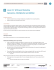Lesson 3 2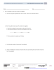Lesson 5 2